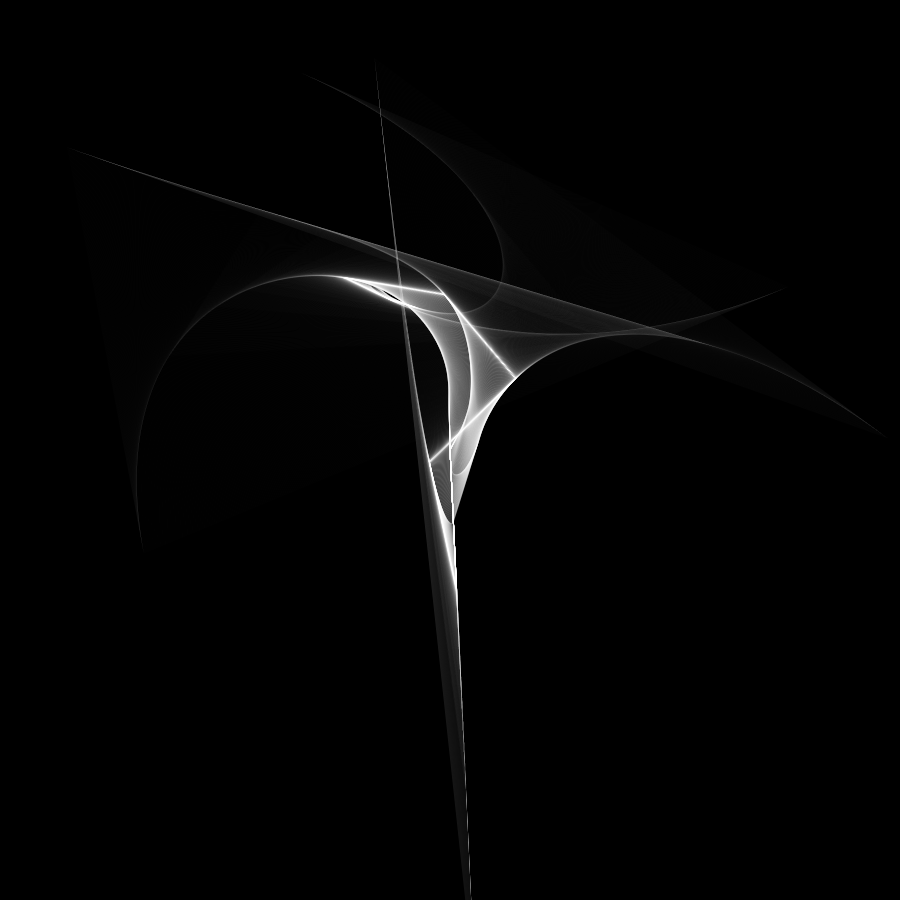# Stacked ClockClick here to view.

This is one of my favorite experiments. I had the original idea during a vacation to Hawaii. Basically, I wanted to make a clock where the hands were connected end-to-tip instead of all being connected to the center of the clock, making a sort of time arm (second hand starting where minute hand ended, etc). I made a version of that using Rainmeter on my desktop for a while.

Sometime later, I was experimenting with curves and lines, and had another idea. I had seen a chain simulation as a Paper.js demo in which as you moved the mouse, each successive link in the chain moved toward the previous link, dragging itself link-by-link. Instead of connecting the points to each other and moving in small amounts, I made the first point jump to a new position, and then drew out the paths each point took toward its neighbor. This generated a smooth curve that followed the straight path of the first point.

I then extended this even further by extrapolating in the other direction. I knew the relationship between the motion of a point and the motion of the next neighbor point: the neighbor point moved toward the previous one until it got to a certain distance away. If you only knew the motion of the neighbor point, you could still figure out the location of the original point, because you know it must be in the direction of the neighbor point's motion and that distance further. I thus could extend the curve past where the initial point was, creating a sweeping arc that passed through the initial point.

I then simply applied this process to the end-to-tip clock, moving the initial point along the hands and then letting the other points follow. The result is a strange, curving structure that is different each time you see it.

If you want to read it, the hands are arranged as follows, starting from the center: Month hand (completes a revolution once per year), Day hand (one revolution per month), Weekday hand (one revolution per week), 24 hour hand, 12 hour hand, minute hand, and second hand.